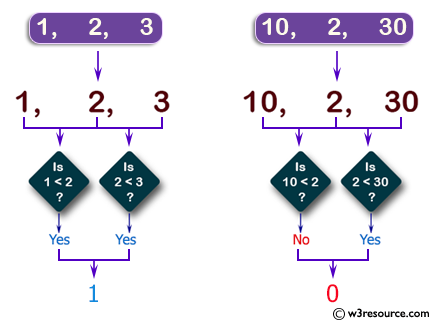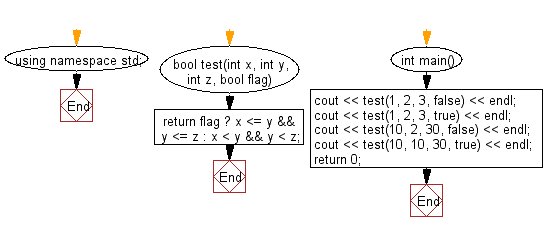﻿ C++ : Check if three numbers are in strict increasing order# C++ Exercises: Check if three given numbers are in strict increasing order

## C++ Basic Algorithm: Exercise-46 with Solution

Write a C++ program to check if three given numbers are in strict increasing order, such as 4 7 15, or 45, 56, 67, but not 4 ,5, 8 or 6, 6, 8. However,if a fourth parameter is true, equality is allowed, such as 6, 6, 8 or 7, 7, 7.

Sample Solution:

C++ Code :

``````#include <iostream>

using namespace std;

bool test(int x, int y, int z, bool flag)
{
return flag ? x <= y && y <= z : x < y && y < z;
}

int main()
{
cout << test(1, 2, 3, false) << endl;
cout << test(1, 2, 3, true) << endl;
cout << test(10, 2, 30, false) << endl;
cout << test(10, 10, 30, true) << endl;
return 0;
}
``````

Sample Output:

```1
1
0
1
```

Pictorial Presentation:Flowchart:C++ Code Editor: